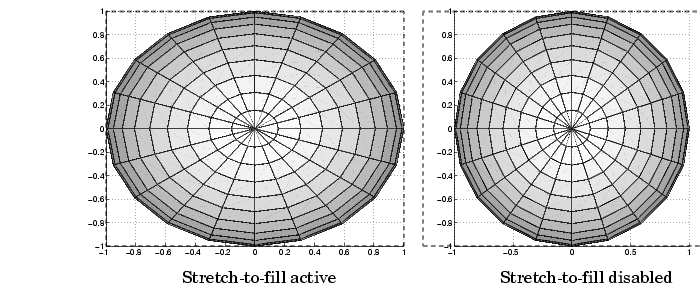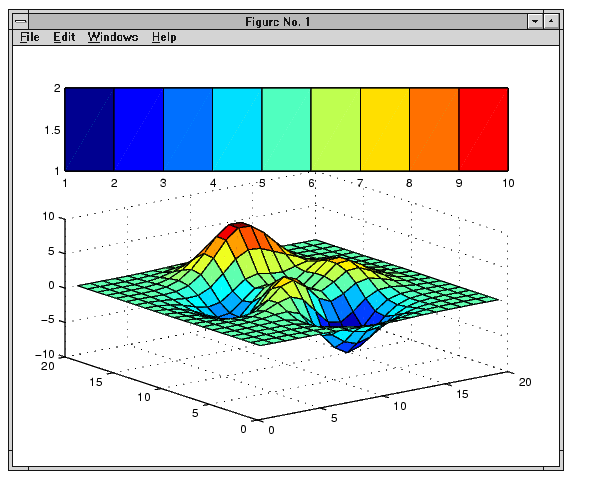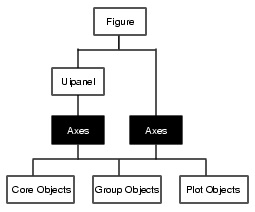MATLAB Function Referenceaxes

Create axes graphics object

Syntax

• ```axes
axes('PropertyName',PropertyValue,...)
axes(h)
h = axes(...)
```

Description

`axes` is the low-level function for creating axes graphics objects.

```axes ``` creates an axes graphics object in the current figure using default property values.

```axes('PropertyName',PropertyValue,...) ``` creates an axes object having the specified property values. MATLAB uses default values for any properties that you do not explicitly define as arguments.

```axes(h) ``` makes existing axes `h` the current axes. It also makes `h` the first axes listed in the figure's `Children` property and sets the figure's `CurrentAxes` property to `h`. The current axes is the target for functions that draw image, line, patch, surface, and text graphics objects.

```h = axes(...) ``` returns the handle of the created axes object.

Remarks

MATLAB automatically creates an axes, if one does not already exist, when you issue a command that creates a graph.

The `axes` function accepts property name/property value pairs, structure arrays, and cell arrays as input arguments (see the `set` and `get` commands for examples of how to specify these data types). These properties, which control various aspects of the axes object, are described in the Axes Properties section.

Use the `set` function to modify the properties of an existing axes or the `get` function to query the current values of axes properties. Use the `gca` command to obtain the handle of the current axes.

The `axis` (not `axes`) function provides simplified access to commonly used properties that control the scaling and appearance of axes.

While the basic purpose of an axes object is to provide a coordinate system for plotted data, axes properties provide considerable control over the way MATLAB displays data.

Stretch-to-Fill

By default, MATLAB stretches the axes to fill the axes position rectangle (the rectangle defined by the last two elements in the `Position` property). This results in graphs that use the available space in the rectangle. However, some 3-D graphs (such as a sphere) appear distorted because of this stretching, and are better viewed with a specific three-dimensional aspect ratio.

Stretch-to-fill is active when the `DataAspectRatioMode`, PlotBoxAspectRatioMode, and CameraViewAngleMode are all auto (the default). However, stretch-to-fill is turned off when the `DataAspectRatio`, PlotBoxAspectRatio, or CameraViewAngle is user-specified, or when one or more of the corresponding modes is set to manual (which happens automatically when you set the corresponding property value).

This picture shows the same sphere displayed both with and without the stretch-to-fill. The dotted lines show the axes rectangle.When stretch-to-fill is disabled, MATLAB sets the size of the axes to be as large as possible within the constraints imposed by the `Position` rectangle without introducing distortion. In the picture above, the height of the rectangle constrains the axes size.

Examples

Zooming

Zoom in using aspect ratio and limits:

• ```sphere
set(gca,'DataAspectRatio',[1 1 1],...
'PlotBoxAspectRatio',[1 1 1],'ZLim',[-0.6 0.6])
```

Zoom in and out using the `CameraViewAngle`:

• ```sphere
set(gca,'CameraViewAngle',get(gca,'CameraViewAngle')-5)
set(gca,'CameraViewAngle',get(gca,'CameraViewAngle')+5)
```

Note that both examples disable the MATLAB stretch-to-fill behavior.

Positioning the Axes

The axes `Position` property enables you to define the location of the axes within the figure window. For example,

• ``` h = axes('Position',position_rectangle)
```

creates an axes object at the specified position within the current figure and returns a handle to it. Specify the location and size of the axes with a rectangle defined by a four-element vector,

• ```position_rectangle = [left, bottom, width, height];
```

The `left` and `bottom` elements of this vector define the distance from the lower left corner of the figure to the lower left corner of the rectangle. The `width` and `height` elements define the dimensions of the rectangle. You specify these values in units determined by the `Units` property. By default, MATLAB uses normalized units where (0,0) is the lower left corner and (1.0,1.0) is the upper right corner of the figure window.

You can define multiple axes in a single figure window:

• ```axes('position',[.1  .1  .8  .6])
mesh(peaks(20));
axes('position',[.1  .7  .8  .2])
pcolor([1:10;1:10]);
```

In this example, the first plot occupies the bottom two-thirds of the figure, and the second occupies the top third.Object HierarchySetting Default Properties

You can set default axes properties on the figure and root levels:

• ```set(0,'DefaultAxesPropertyName',PropertyValue,...)
set(gcf,'DefaultAxesPropertyName',PropertyValue,...)
```

where `PropertyName` is the name of the axes property and `PropertyValue` is the value you are specifying. Use `set` and `get` to access axes properties.

See Also

`axis`, `cla`, `clf`, `figure`, `gca`, `grid`, `subplot`, `title`, `xlabel`, `ylabel`, `zlabel`, `view`

Axes Operations for related functions

Axes Properties for more examples

See Types of Graphics Objects for information on core, group, plot, and annotation objects.aviread Axes Properties© 1994-2005 The MathWorks, Inc.